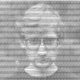I’m sure there’s a better way out there, but I struggled to find a way to dissolve polygons that touched/overlapped each other (the special case being buffers). For example,  using the osmdata package, we can download the polygons representing hospital buildings in Bern, Switzerland.

```library(osmdata)
library(rgdal) ; library(maptools) ; library(rgeos)

q0 <- opq(bbox = "Bern, Switzerland", timeout = 60)
q1 <- add_osm_feature(q0, key = 'building', value = "hospital")
x <- osmdata_sp(q1)

library(leaflet)

spChFIDs(x\$osm_polygons) <- 1:nrow(x\$osm_polygons@data)
cent <- gCentroid(x\$osm_polygons, byid = TRUE)

Here we plot the building centroids.Each point represents a hospital building. We don’t particularly care about the buildings themselves though. We want to create hospitals. To do so, we try a 150m buffer around each centroid.

```buff <- gBuffer(cent, byid = TRUE, width = 0.0015)We then want to merge the buffers into, in this case, four groups. This is the point that doesn’t seem to be implemented anywhere that I could see (I also tried QGIS but that just created one big feature, rather than many small ones). My approach is to get the unique sets of intersections, add them as a variable to the buffer and unify the polygons.

```buff <- SpatialPolygonsDataFrame(buff, data.frame(row.names = names(buff), n = 1:length(buff)))
gt <- gIntersects(buff, byid = TRUE, returnDense = FALSE)
ut <- unique(gt)
nth <- 1:length(ut)
buff\$n <- 1:nrow(buff)
buff\$nth <- NA
for(i in 1:length(ut)){
x <- ut[[i]]
buff\$nth[x] <- i
}
buffdis <- gUnaryUnion(buff, buff\$nth)As you see, it almost worked. The lower left group is composed of three polygons. Doing the same process again clears it (only code shown). Large jobs might need more iterations (or larger buffers). The final job is to get the hospital centroids.

```gt <- gIntersects(buffdis, byid = TRUE, returnDense = FALSE)
ut <- unique(gt)
nth <- 1:length(ut)
buffdis <- SpatialPolygonsDataFrame(buffdis, data.frame(row.names = names(buffdis), n = 1:length(buffdis)))
buffdis\$nth <- NA
for(i in 1:length(ut)){
x <- ut[[i]]
buffdis\$nth[x] <- i
}
buffdis <- gUnaryUnion(buffdis, buffdis\$nth)

buffcent <- gCentroid(buffdis, byid = TRUE```

From → R, Uncategorized

1.Did you look into using the sf package with st_union? https://r-spatial.github.io/sf/reference/geos_combine.html

•No. I didn’t see that. Thanks for pointing it out.

•This should do:

library(osmdata)
library(rgdal)
library(maptools)
library(rgeos)
library(mapview)
library(sf)

q0 <- opq(bbox = "Bern, Switzerland", timeout = 60)
q1 <- add_osm_feature(q0, key = 'building', value = "hospital")
x % st_cast(“POLYGON”)

mapview(fin_pols)

•WordPress does weird things to code in comments… I think you’ve been another victim…

•2.I’d suggest to have a while statement, since in some cases you will need more then two cycles.

“`
ut 0){
ut <- unique(sapply(ut, function(x)
unique(unlist(ut[sapply(ut, function(y)
any(x %in% y))]))))}
“`

•For some reason it is get my code wrong

ut 0){
ut <- unique(sapply(ut, function(x)
unique(unlist(ut[sapply(ut, function(y)
any(x %in% y))]))))}

•Wow… WordPress is eating my letters.
code here

•I thought something weird was happening 😉

3.Nice post. My 2 cents on an alternative approach to solving this issue

This file contains bidirectional Unicode text that may be interpreted or compiled differently than what appears below. To review, open the file in an editor that reveals hidden Unicode characters.

 library(sp) library(osmdata) library(rgeos) library(leaflet) # Download data q0 <- opq(bbox = "Bern, Switzerland", timeout = 60) q1 <- add_osm_feature(q0, key = 'building', value = "hospital") x <- osmdata_sp(q1) # Calculate polygons entroid and buffer spChFIDs(x\$osm_polygons) <- 1:nrow(x\$osm_polygons@data) cent <- gCentroid(x\$osm_polygons, byid = TRUE) buff <- gBuffer(cent, byid = TRUE, width = 0.0015) # Dissolve, split multypolygon to single polygons and calculate the centroids buffdis <- gUnaryUnion(buff) split_buff <- disaggregate(buffdis) buffcent <- gCentroid(split_buff, byid = TRUE) # Plot the result leaflet(buffcent) %>% addTiles() %>% addPolygons(data = split_buff, col = "red") %>% addCircles()

view raw

merge_sp.R

hosted with ❤ by GitHub

•Thanks. That’s one of the good (or bad?) things with R…many ways to achieve a goal… 🙂